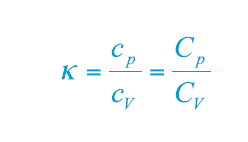Our Solutions
Atlas Copco Rental
Solutions
Atlas Copco Rental
Atlas Copco Rental
Atlas Copco Rental
Atlas Copco Rental
Atlas Copco Rental
Compressors
Solutions
Compressors
Compressors
Compressors
Compressors
Industrial Tools & Solutions
Solutions
Industrial Tools & Solutions
Industrial Tools & Solutions
Industrial Tools & Solutions
Industrial Tools & Solutions
Power Equipment
Solutions
Power Equipment
Power Equipment
Power Equipment
Vacuum solutions
Solutions
Vacuum solutions

# Measuring Pressure, Temperature and Thermal Capacity

After learning about the basics of physics here, you might want to know more concerning the physical units used to measure different aspects of matter. This can be very helpful when dealing with compressed air. In this article, we will explain the basics of measuring pressure, temperature and thermal capacity.

## What is pressure and how do we measure it?

The force on a square centimeter area of an air column, which runs from sea level to the edge of the atmosphere, is about 10.13 N. Therefore, the absolute atmospheric pressure at sea level is approx. 10.13 x 104 N per square meter, which is equal to 10.13 x 104 Pa (Pascal, the SI unit for pressure). Expressed in another frequently used unit: 1 bar = 1 x 105 Pa. The higher you are above (or below) sea level, the lower (or higher) the atmospheric pressure.

## How do we measure temperature?

The temperature of a gas is more difficult to define clearly. Temperature is a measure of the kinetic energy in molecules. Molecules move more rapidly the higher the temperature, and movement completely ceases at a temperature of absolute zero. The Kelvin (K) scale is based on this phenomenon, but otherwise is graduated in the same manner as the centigrade or Celsius (C) scale:

T = t + 273.2
T = absolute temperature (K)
t = centigrade temperature c°

## How is heat capacity measured?

Heat is a form of energy, represented by the kinetic energy of the disordered molecules of a substance. The thermal capacity (also called heat capacity) of an object refers to the quantity of heat required to produce a unit change of temperature (1K), and is expressed in J/K. The specific heat or specific thermal capacity of a substance is more commonly used, and refers to the quantity of heat required to produce a unit change of temperature (1K) in a unit mass of substance (1 kg). cp = specific heat at constant pressure cV = specific heat at constant volume Cp = molar specific heat at constant pressure CV = molar specific heat at constant volume The specific heat at constant pressure is always greater than the specific heat at constant volume. The specific heat for a substance is not a constant, but rises, in general, as the temperature rises. For practical purposes, a mean value may be used. For liquids and solid substances cp ≈ cV ≈ c. To heat a mass flow (m) from temperature t1 to t2 will then require: P = m x c x (T2 -T1) P = heat power (W) m= mass flow (kg/s) c = specific heat (J/kg x K) T = temperature (K)

The explanation as to why cp is greater than cV is the expansion work that the gas at a constant pressure must perform. The ratio between cp and cV is called the isentropic exponent or adiabatic exponent, К, and is a function of the number of atoms in the molecules of the substance.### Related articles

#### The Structure and Different Stages of Matter

To understand the workings of compressed air, a basic introduction to physics can come a long way. We start by explaining the structure and four different states of matter. Learn more.

#### Measuring Work, Power and Volume Rate of Flow

To understand the workings of compressed air, a basic introduction to physics can come a long way. Let us take a look at the physical units for measuring work, power and volume rate of flow. Learn more.

#### An Introduction to Thermodynamics: Main Principles and Gas Laws

To understand the workings of compressed air, a basic introduction to physics can come a long way. Learn more about thermodynamics and how they are vital in understanding how air compressors work.